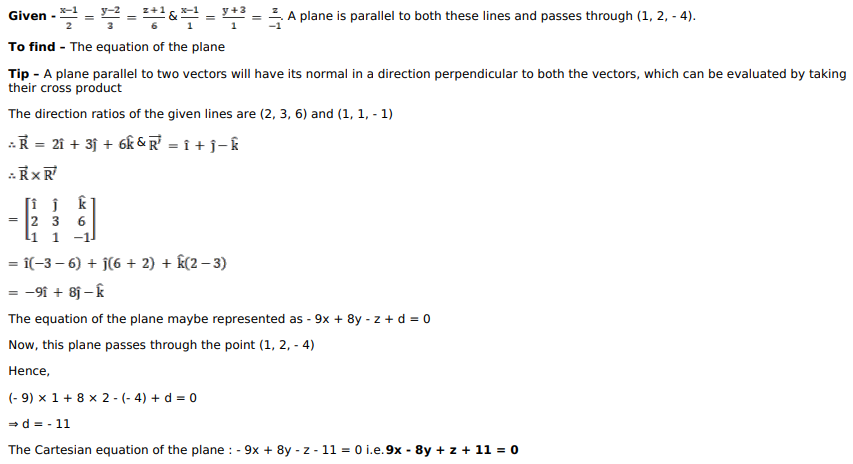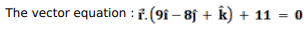# Find the Cartesian and vector equations of a plane passing through the point

Question:

Find the Cartesian and vector equations of a plane passing through the point $(1,2,-4)$ and parallel to the lines $\frac{x-1}{2}=\frac{y-2}{3}=\frac{z+1}{6}$ and $\frac{x-1}{1}=\frac{y+3}{1}=\frac{z}{-1}$

Solution: